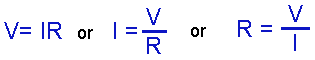# Ohm’s Law

• After studying this section, you should be able to:
• Describe Ohm’s Law involving metalic conductors:
• • Resistance, Voltage & Current.
• Define:
• The Ohm, Ampere & Volt.

## Ohms, Volts & Amperes.

The resistance of a conductor is measured in Ohms and the Ohm is a unit named after the German physicist George Simon Ohm (1787-1854) who was the first to show the relationship between resistance, current and voltage. In doing so he devised his law which shows the inter-relationship between the three basic electrical properties of resistance, voltage and current. It demonstrates one of the most important relationships in electrical and electronic engineering.

Ohm´s Law states that: "In metallic conductors at a constant temperature and in a zero magnetic field, the current flowing is proportional to the voltage across the ends of the conductor, and is inversely proportional to the resistance of the conductor."

In simple terms, provided that the temperature is constant and the electrical circuit is not influenced by magnetic fields, then:

• With a circuit of constant resistance, the greater the voltage applied to a circuit, the more current will flow.

• With a constant voltage applied, the greater the resistance of the circuit, the less current will flow.

Notice that Ohm´s law states "In metallic conductors" This means that the law holds good for most materials that are metal, but not all. Tungsten for example, used for the glowing filaments of light bulbs has a resistance that changes with the temperature of the filament, hence the reference in Ohm´s Law to ‘at a constant temperature’. There are also components used in electronics that have a non-linear relationship between the three electrical properties of voltage, current and resistance, but these can be described by different formulae. For the majority of circuits or components, which can be described by Ohm´s Law:

Rather than trying to remember the whole of Ohm´s law, the three electrical properties of voltage, current and resistance by single letters:

Resistance is indicated by the letter R and is measured in units of Ohms, which have the symbol Ω (Greek capital O).

Voltage is indicated by the letter V (or sometimes E, an abbreviation for Electromotive Force) and is measured in units of Volts, which have the symbol V.

Current is given the letter I (not C as this is used for Capacitance) and is measured in units of Amperes (often shortened to Amps), which have the symbol A.

By using the letters V, I and R to express the relationships defined in Ohms Law gives three simple formulae:Each of which shows how to find the value of any one of these quantities in a circuit, provided the other two are known. For example, to find the voltage V (in Volts) across a resistor, simply multiply the current I (in Amperes) through the resistor by the value of the resistor R (in Ohms).Note that when using these formulae the values of V I and R written into the formula must be in its BASIC UNIT i.e. VOLTS (not millivolts) Ohms (not kilohms) and AMPERES (not micro Amperes )etc.

Briefly 15KΩ (kilohms) is entered as 15 EXP 03 and 25mA (milliAmperes) is entered as 25 EXP -03 etc. This is easiest to do using a scientific calculator.

How to use your calculator with the engineering notation used extensively in electronics is explained in our free booklet entitled "Maths Tips" Download it from our Download page.

## Defining The Ohm, Ampere & Volt

### 1 OHM

Can be defined as "The amount of resistance that will produce a potential difference (p.d.) or voltage of 1 Volt across it when a current of 1 Ampere is flowing through it."

### 1 AMPERE

Can be defined as "The amount of current which, when flowing through a resistance of 1 Ohm will produce a potential difference of 1 Volt across the resistance."

(Although more useful definitions of an ampere are available)

### 1 VOLT

Can be defined as "The difference in potential (voltage) produced across a resistance of 1 Ohm through which a current of 1 Ampere is flowing."

These definitions relate Volts, Amperes and Ohms within the quantities described in Ohm´s Law, but alternative definitions using other quantities can also be used.

Top of Page.>